# Gauss Linear Model

The Gauss statistical model says that the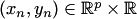are generated as follows:

• there are no restrictions on the way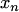are generated;
• given, the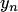are generated from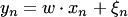, where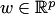is a vector of parameters,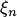is distributed as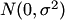, and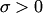is another parameter.

See Section 8.5 of Vovk et al. (2005) and Vovk et al. (2009) for the formulation of this model as an on-line compression model.

The most basic version of this model is where there are no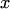s, and the model is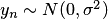. The summary ofis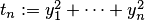and the Gauss repetitive structure postulates that the distribution ofis uniform on the sphere of radius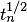. Borel (1914) noticed that the Gauss statistical model (used by Maxwell as a model in statistical physics) is equivalent to the Gauss repetitive structure (used for a similar purpose by Gibbs). For further historical comments, see Vovk et al. (2005), Section 8.8, and Diaconis and Freedman (1987), Section 6.

Bibliography

• Persi Diaconis and David Freedman (1987). A dozen de Finetti-style results in search of a theory. Annales de l'Institut Henri Poincare B 23:397-423.
• Vladimir Vovk, Alexander Gammerman and Glenn Shafer (2005). Algorithmic learning in a random world. Springer, New York.
• Vladimir Vovk, Ilia Nouretdinov, and Alexander Gammerman (2009). On-line predictive linear regression. Annals of Statistics 37:1566-1590.